Anúncio

# 0Assignment Nos.1,2,3,4.docx

19 de Aug de 2022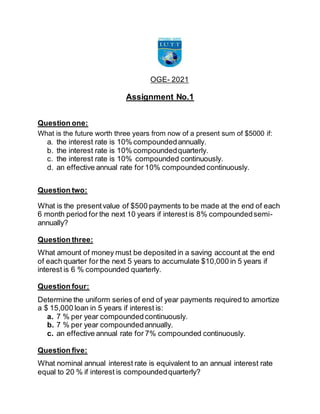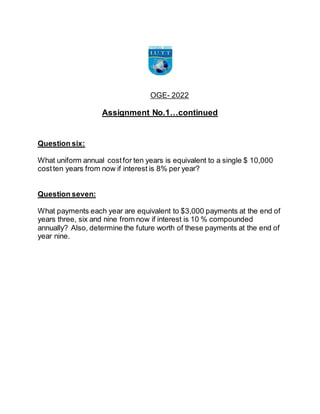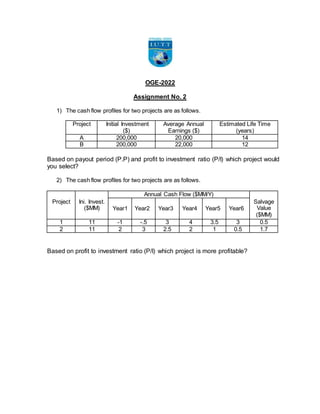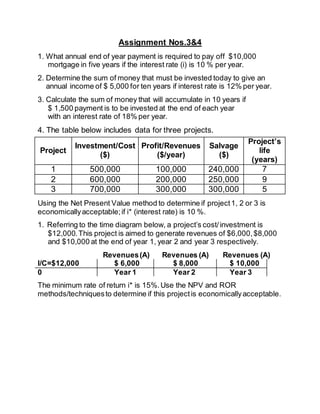Próximos SlideShares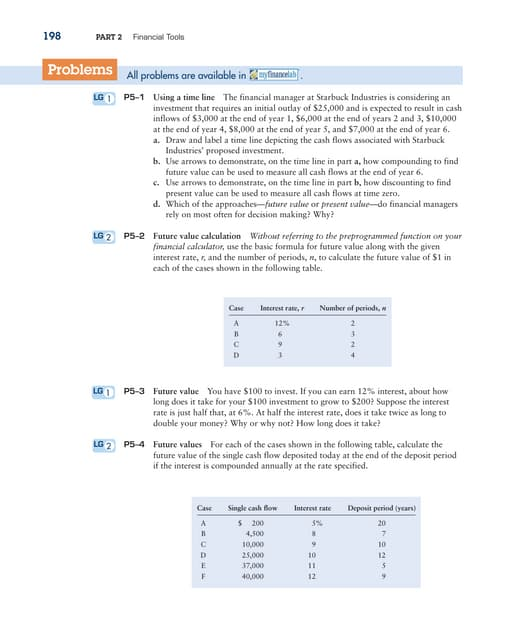TVM Questions.pdf
Carregando em ... 3
1 de 4
Anúncio

### 0Assignment Nos.1,2,3,4.docx

1. OGE- 2021 Assignment No.1 Question one: What is the future worth three years from now of a present sum of \$5000 if: a. the interest rate is 10% compoundedannually. b. the interest rate is 10% compoundedquarterly. c. the interest rate is 10% compounded continuously. d. an effective annual rate for 10% compounded continuously. Question two: What is the presentvalue of \$500 payments to be made at the end of each 6 month period for the next 10 years if interest is 8% compoundedsemi- annually? Question three: What amount of money must be deposited in a saving account at the end of each quarter for the next 5 years to accumulate \$10,000 in 5 years if interest is 6 % compounded quarterly. Question four: Determine the uniform series of end of year payments required to amortize a \$ 15,000 loan in 5 years if interest is: a. 7 % per year compounded continuously. b. 7 % per year compoundedannually. c. an effective annual rate for 7% compounded continuously. Question five: What nominal annual interest rate is equivalent to an annual interest rate equal to 20 % if interest is compoundedquarterly?
2. OGE- 2022 Assignment No.1…continued Question six: What uniform annual costfor ten years is equivalent to a single \$ 10,000 costten years from now if interest is 8% per year? Question seven: What payments each year are equivalent to \$3,000 payments at the end of years three, six and nine from now if interest is 10 % compounded annually? Also, determine the future worth of these payments at the end of year nine.
3. OGE-2022 Assignment No. 2 1) The cash flow profiles for two projects are as follows. Project Initial Investment (\$) Average Annual Earnings (\$) Estimated Life Time (years) A 200,000 20,000 14 B 200,000 22,000 12 Based on payout period (P.P) and profit to investment ratio (P/I) which project would you select? 2) The cash flow profiles for two projects are as follows. Project Ini. Invest. (\$MM) Annual Cash Flow (\$MM/Y) Salvage Value (\$MM) Year1 Year2 Year3 Year4 Year5 Year6 1 11 -1 -.5 3 4 3.5 3 0.5 2 11 2 3 2.5 2 1 0.5 1.7 Based on profit to investment ratio (P/I) which project is more profitable?
4. Assignment Nos.3&4 1. What annual end of year payment is required to pay off \$10,000 mortgage in five years if the interest rate (i) is 10 % per year. 2. Determine the sum of money that must be invested today to give an annual income of \$ 5,000 for ten years if interest rate is 12% per year. 3. Calculate the sum of money that will accumulate in 10 years if \$ 1,500 payment is to be invested at the end of each year with an interest rate of 18% per year. 4. The table below includes data for three projects. Project Investment/Cost (\$) Profit/Revenues (\$/year) Salvage (\$) Project’s life (years) 1 500,000 100,000 240,000 7 2 600,000 200,000 250,000 9 3 700,000 300,000 300,000 5 Using the Net Present Value method to determine if project1, 2 or 3 is economicallyacceptable;if i* (interest rate) is 10 %. 1. Referring to the time diagram below, a project’s cost/investment is \$12,000.This project is aimed to generate revenues of \$6,000,\$8,000 and \$10,000 at the end of year 1, year 2 and year 3 respectively. Revenues(A) Revenues (A) Revenues (A) I/C=\$12,000 \$ 6,000 \$ 8,000 \$ 10,000 0 Year 1 Year 2 Year 3 The minimum rate of return i* is 15%.Use the NPV and ROR methods/techniquesto determine if this projectis economicallyacceptable.
Anúncio